# Check if a string is a subsequence of another string ( using Stacks )

• Difficulty Level : Easy
• Last Updated : 10 Jun, 2021

Given a string S, the task is to check if the string target is a subsequence of string S or not, using a Stack.

Examples:

Input: S = ”KOTTAYAM”, target = ”KOTA”
Output: Yes
Explanation: “KOTA” is a subsequence of “KOTTAYAM”.

Input: S = ”GEEKSFORGEEKS”, target =”FORFOR”
Output: No

Approach: Follow the steps to solve the problem: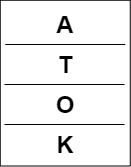target pushed into the stack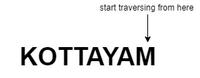Traversing in S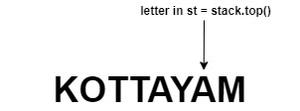Traversing in S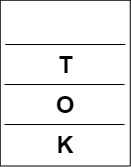Popping from stack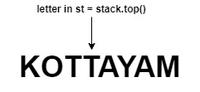Traversing in S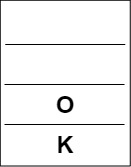Popping from stack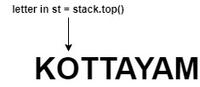Traversing in st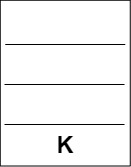Popping from stack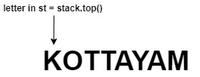Traversing in S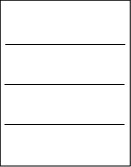Stack becomes empty

Below is the implementation of the above approach:

## C++

 `// C++ Program for the above approach`   `#include ` `using` `namespace` `std;`   `// Function to check if target` `// is a subsequence of string S` `void` `checkforSubsequence(string S,` `                         ``string target)` `{`   `    ``// Declare a stack` `    ``stack<``char``> s;`   `    ``// Push the characters of` `    ``// target into the stack` `    ``for` `(``int` `i = 0; i < target.size(); i++) {` `        ``s.push(target[i]);` `    ``}`   `    ``// Traverse the string S in reverse` `    ``for` `(``int` `i = (``int``)S.size() - 1; i >= 0; i--) {`   `        ``// If the stack is empty` `        ``if` `(s.empty()) {`   `            ``cout << ``"Yes"` `<< endl;` `            ``return``;` `        ``}`   `        ``// if S[i] is same as the` `        ``// top of the stack` `        ``if` `(S[i] == s.top()) {`   `            ``// Pop the top of stack` `            ``s.pop();` `        ``}` `    ``}`   `    ``// Stack s is empty` `    ``if` `(s.empty())` `        ``cout << ``"Yes"` `<< endl;` `    ``else` `        ``cout << ``"No"` `<< endl;` `}`   `// Driver Code` `int` `main()` `{` `    ``string S = ``"KOTTAYAM"``;` `    ``string target = ``"KOTA"``;`   `    ``checkforSubsequence(S, target);`   `    ``return` `0;` `}`

## Java

 `// Java approach for the above approach` `import` `java.util.Stack;`   `public` `class` `GFG {`   `    ``// Function to check if target` `    ``// is a subsequence of string S` `    ``static` `void` `checkforSubsequence(String S, String target)` `    ``{`   `        ``// Declare a stack` `        ``Stack s = ``new` `Stack<>();`   `        ``// Push the characters of` `        ``// target into the stack` `        ``for` `(``int` `i = ``0``; i < target.length(); i++) {` `            ``s.push(target.charAt(i));` `        ``}`   `        ``// Traverse the string S in reverse` `        ``for` `(``int` `i = (``int``)S.length() - ``1``; i >= ``0``; i--) {`   `            ``// If the stack is empty` `            ``if` `(s.empty()) {`   `                ``System.out.println(``"Yes"``);` `                ``return``;` `            ``}`   `            ``// if S[i] is same as the` `            ``// top of the stack` `            ``if` `(S.charAt(i) == s.peek()) {`   `                ``// Pop the top of stack` `                ``s.pop();` `            ``}` `        ``}`   `        ``// Stack s is empty` `        ``if` `(s.empty())` `            ``System.out.println(``"Yes"``);` `        ``else` `            ``System.out.println(``"No"``);` `    ``}`   `    ``// Driver Code` `    ``public` `static` `void` `main(String[] args)` `    ``{` `        ``String S = ``"KOTTAYAM"``;` `        ``String target = ``"KOTA"``;`   `        ``checkforSubsequence(S, target);` `    ``}` `}`   `// This code is contributed by abhinavjain194`

## Python3

 `# Python3 program for the above approach`   `# Function to check if target` `# is a subsequence of string S` `def` `checkforSubsequence(S, target):`   `    ``# Declare a stack` `    ``s ``=` `[]`   `    ``# Push the characters of` `    ``# target into the stack` `    ``for` `i ``in` `range``(``len``(target)):` `        ``s.append(target[i])`   `    ``# Traverse the string S in reverse` `    ``for` `i ``in` `range``(``len``(S) ``-` `1``, ``-``1``, ``-``1``):`   `        ``# If the stack is empty` `        ``if` `(``len``(s) ``=``=` `0``):` `            ``print``(``"Yes"``)` `            ``return`   `        ``# If S[i] is same as the` `        ``# top of the stack` `        ``if` `(S[i] ``=``=` `s[``-``1``]):`   `            ``# Pop the top of stack` `            ``s.pop()`   `    ``# Stack s is empty` `    ``if` `(``len``(s) ``=``=` `0``):` `        ``print``(``"Yes"``)` `    ``else``:` `        ``print``(``"No"``)`   `# Driver Code` `if` `__name__ ``=``=` `"__main__"``:`   `    ``S ``=` `"KOTTAYAM"` `    ``target ``=` `"KOTA"`   `    ``checkforSubsequence(S, target)` `    `  `# This code is contributed by ukasp`

## C#

 `// C# approach for the above approach` `using` `System;` `using` `System.Collections.Generic;`   `class` `GFG{`   `// Function to check if target` `// is a subsequence of string S` `static` `void` `checkforSubsequence(String S, ` `                                ``String target)` `{` `    `  `    ``// Declare a stack` `    ``Stack<``char``> s = ``new` `Stack<``char``>();`   `    ``// Push the characters of` `    ``// target into the stack` `    ``for``(``int` `i = 0; i < target.Length; i++)` `    ``{` `        ``s.Push(target[i]);` `    ``}`   `    ``// Traverse the string S in reverse` `    ``for``(``int` `i = (``int``)S.Length - 1; i >= 0; i--) ` `    ``{`   `        ``// If the stack is empty` `        ``if` `(s.Count == 0) ` `        ``{` `            ``Console.WriteLine(``"Yes"``);` `            ``return``;` `        ``}`   `        ``// If S[i] is same as the` `        ``// top of the stack` `        ``if` `(S[i] == s.Peek())` `        ``{` `            `  `            ``// Pop the top of stack` `            ``s.Pop();` `        ``}` `    ``}`   `    ``// Stack s is empty` `    ``if` `(s.Count == 0)` `        ``Console.WriteLine(``"Yes"``);` `    ``else` `        ``Console.WriteLine(``"No"``);` `}`   `// Driver Code` `public` `static` `void` `Main(String[] args)` `{` `    ``String S = ``"KOTTAYAM"``;` `    ``String target = ``"KOTA"``;`   `    ``checkforSubsequence(S, target);` `}` `}`   `// This code is contributed by shikhasingrajput`

## Javascript

 ``

Output:

`Yes`

Time Complexity : O(N)
Auxiliary Space : O(N)

My Personal Notes arrow_drop_up
Recommended Articles
Page :